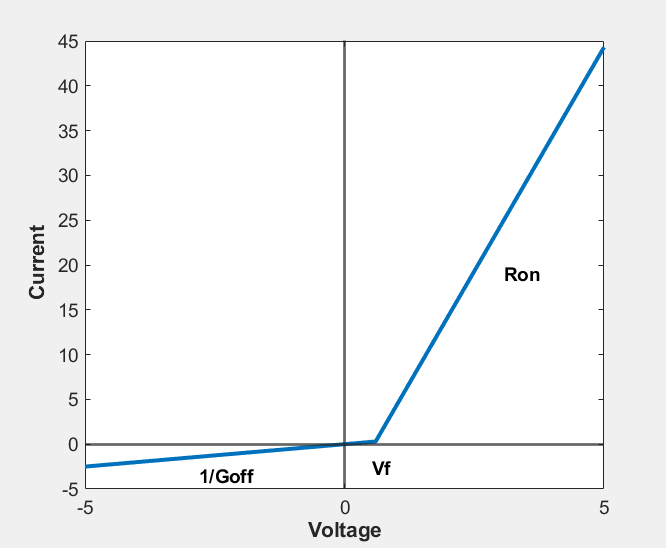# Diode

Piecewise linear diode in electrical systems

•Libraries:
Simscape / Foundation Library / Electrical / Electrical Elements

## Description

The Diode block models a piecewise linear diode. If the voltage across the diode is bigger than the Forward voltage parameter value, then the diode behaves like a linear resistor with low resistance, given by the On resistance parameter value, plus a constant series voltage source. If the voltage across the diode is less than the forward voltage, then the diode behaves like a linear resistor with low conductance given by the Off conductance parameter value.When forward biased, the diode behavior is described with the equations:

`$\begin{array}{l}v=i\cdot {R}_{on}+{V}_{source},\\ {V}_{source}=Vf\left(1-{R}_{on}{G}_{off}\right),\end{array}$`

where:

• v is the voltage.

• i is the current.

• Vsource is the voltage of the series voltage source.

• Vf is the forward voltage.

• Ron is the resistance of the forward-biased diode.

• Goff is the conductance of the reverse-biased diode.

The reverse behavior is given byv = i/Goff.

The Ron.Goff term ensures that there is no discontinuity in the diode current when the diode is switching between the on and off states.

### Variables

To set the priority and initial target values for the block variables prior to simulation, use the Initial Targets section in the block dialog box or Property Inspector. For more information, see Set Priority and Initial Target for Block Variables.

Nominal values provide a way to specify the expected magnitude of a variable in a model. Using system scaling based on nominal values increases the simulation robustness. Nominal values can come from different sources, one of which is the Nominal Values section in the block dialog box or Property Inspector. For more information, see Modify Nominal Values for a Block Variable.

## Ports

### Conserving

expand all

Electrical conserving port associated with the diode positive terminal.

Electrical conserving port associated with the diode negative terminal.

## Parameters

expand all

Minimum voltage that needs to be applied for the diode to become forward biased.

The resistance of the forward-biased diode.

The conductance of the reverse-biased diode.

## Version History

Introduced in R2007a

expand all